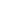Flat 50% off

Ends in

###### Linear Inequations, CBSE 11th Mathematics in English
3,938 views###### Linear Inequations, CBSE 11th Mathematics in English

Published on 05, Aug 2019

Watch video lecture about Binomial distribution simultaneous linear inequations in two variables from chapter Linear Inequations of Mathematics, online video lecture for CBSE class 11th students, prepared by the best-experienced faculty of Misostudy (https://www.misostudy.com). The graph of an inequality in two variables is the set of points that represents all solutions to inequality. A linear inequality divides the coordinate plane into two halves by a boundary line where one half represents the solutions of the inequality. In this online video lecture, we will discuss the Theory and Problem Solving of Linear Inequations from Mathematics for the preparation of CBSE 2020 exam. Misostudy's courses will help students to boost CBSE preparation to perform best in exams and achieve a good rank.